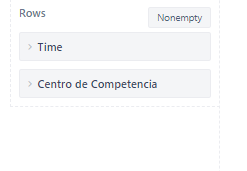# Error when using sparklines in tables with "others" value

HI!

I get an error when I try to use sparklines with the All Others data
I can use it with Gauge but with table it gives that error

Any ideas?

Thanks
Walter

This is a known issue that was fixed in the eazyBI version 6.4.0 ( released Oct 29, 2021 ).

A workaround for older versions would be to define a new calculated measure with the following formula:

``````CASE WHEN [Issue Type].CurrentMember.Name <> '\$total_aggregate' AND
[Issue Type].CurrentMember.Name <> '\$all_others'
THEN
[Measures].[Ultimos 6 periodos]
END
``````

Replace the [Issue Type] dimension with the Dimension you are using in the Rows section.

​Best regards,
​Nauris / eazyBI support

1 Like

I’ve tried your recommendation but with a little tweak.

``````CASE WHEN [Issue Type].CurrentMember.Name <> '\$total_aggregate' AND
[Centro de Competencia].CurrentMember.Name <> '\$all_others' --centro de competencia is the one in the rows, but Time dimension is also there.
THEN
[Measures].[Tickets creados] -- this means "issues created"
END
``````But got the same error.

Could it be due to my tweaks?

In your case, since you are using the “All others” option on the Time dimension, you should use the Time dimension in the formula as well:

``````CASE WHEN [Time].CurrentHierarchyMember.Name <> '\$total_aggregate' AND
[Time].CurrentHierarchyMember.Name <> '\$all_others'
THEN
[Measures].[Tickets creados] -- this means "issues created"
END
``````

Hi @nauris.malitis
How should be the formula?

Did you manage to update the eazyBI app?

If not then you can test this formula:

``````CASE WHEN [Time].CurrentHierarchyMember.Name <> '\$total_aggregate' AND
[Time].CurrentHierarchyMember.Name <> '\$all_others'
THEN
[Measures].[Tickets creados] -- this means "issues created"
END
``````

​Best regards,
​Nauris